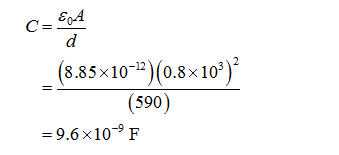# Lightning As a crude model for lightning, consider the ground to be one plate of a parallel-plate capacitor and a cloud at an altitude of 590 m to be the other plate. Assume the surface area of the cloud to be the same as the area of a square that is 0.80 km on a side. (Dielectric strength of air is 3.0×106V/m)What is the capacitance of this capacitor?How much charge can the cloud hold before the dielectric strength of the air is exceeded and a spark (lightning) results?

Question
67 views

Lightning As a crude model for lightning, consider the ground to be one plate of a parallel-plate capacitor and a cloud at an altitude of 590 m to be the other plate. Assume the surface area of the cloud to be the same as the area of a square that is 0.80 km on a side. (Dielectric strength of air is 3.0×106V/m)

What is the capacitance of this capacitor?

How much charge can the cloud hold before the dielectric strength of the air is exceeded and a spark (lightning) results?

check_circle

Step 1

The required capacitance is,...

### Want to see the full answer?

See Solution

#### Want to see this answer and more?

Solutions are written by subject experts who are available 24/7. Questions are typically answered within 1 hour.*

See Solution
*Response times may vary by subject and question.
Tagged in

### Physics